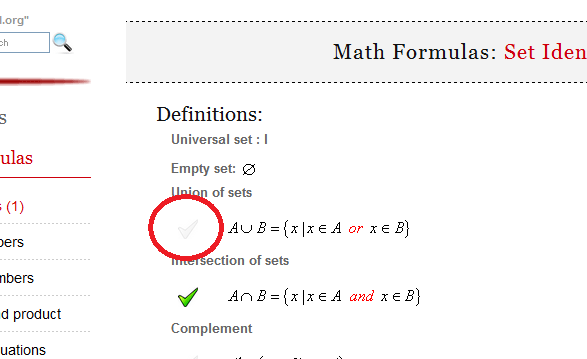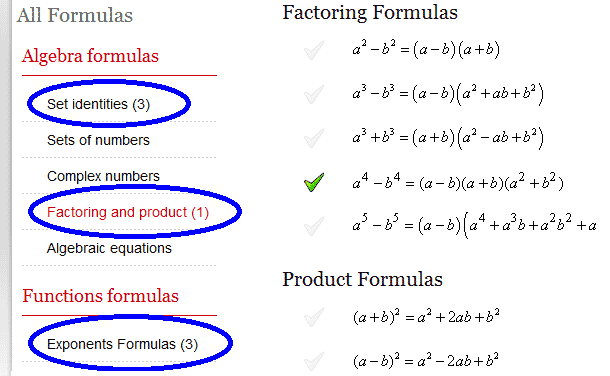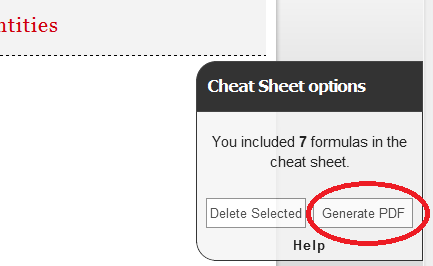Math Calculators, Lessons and Formulas

It is time to solve your math problem

mathportal.org

You included 0 formulas in your custom cheat sheet.

Cheat Sheet options

## How to generate cheat sheet ?

To create cheat sheet first you need to select formulas which you want to include in it. To select formula click at picture next to formula.You can choose formulas from different pages.After you have selected all the formulas which you would like to include in cheat sheet, click the "Generate PDF" button.# Math Formulas: Planes in three dimensions

### Plane forms

Point direction form:

 $$a(x-x_1) + b(y-y_1) + c(z-z_1) = 0$$

where $P(x_1, y_1, z_1)$ lies in the plane, and the direction $(a,b,c)$ is normal to the plane.

General form:

 $$Ax + By + Cz + D = 0$$

where direction $(A,B,C)$ is normal to the plane.

Intercept form:

 $$\frac{x}{a} + \frac{y}{b} + \frac{z}{c} = 1$$

this plane passes through the points $(a,0,0), (0,b,0)$ and $(0,0,c)$.

Three point form:

 $$\begin{vmatrix} x-x_3 & y-y_3 & z-z_3 \\ x_1-x_3 & y_1-y_3 & z_1-z_3 \\ x_2-x_3 & y_2-y_3 & z_2-z_3 \end{vmatrix} = 0$$

Normal form:

 $$x\,\cos \alpha + y\,\cos\beta + z\,\cos\gamma = p$$

Parametric form:

 \begin{aligned} x &= x_1 + a_1\,s + a_2\,t \\ y &= y_1 + b_1\,s + b_2\,t \\ z &= z_1 + c_1\,s + c_2\,t \end{aligned}

where the directions $(a_1, b_1, c_1)$ and $(a_2, b_2, c_2)$ are parallel to the plane.

### Angle between two planes:

The angle between planes $A_1x + B_1y + C_1z + D_1 = 0$ and $A_2x + B_2y + C_2z + D_2 = 0$ is:

 $$\alpha = \arccos \frac{A_1A_2 + B_1B_2 + C_1C_2} {\sqrt{A_1^2 + B_1^2 + C_1^2} \cdot \sqrt{A_2^2 + B_2^2 + C_2^2}}$$

The planes are parallel if and only if

 $$\frac{A_1}{A_2} = \frac{B_1}{B_2} = \frac{C_1}{C_2}$$

### Equation of a plane

The equation of a plane through $P_1(x_1, y_1, z_1)$ and parallel to directions $(a_1, b_1, c_1)$ and $(a_2, b_2, c_2)$ has an equation:

 $$\begin{vmatrix} x-x_1 & y - y_1 & z - z_1 \\ a_1 & b_1 & c_1 \\ a_2 & b_2 & c_2 \end{vmatrix} = 0$$

The equation of a plane through $P_1(x_1, y_1, z_1)$ and$P_1(x_2, y_2, z_2)$), and parallel to direction $(a,b,c)$, has equation

 $$\begin{vmatrix} x-x_1 & y - y_1 & z - z_1 \\ x_2-x_1 & y_2-y_1 & z_2-z_1 \\ a & b & c \end{vmatrix} = 0$$

The equation of a plane through $P_1(x_1, y_1, z_1)$ , $P_2(x_2, y_2, z_2)$ and $P_3(x_3, y_3, z_3)$ , has equation

 $$\begin{vmatrix} x-x_1 & y - y_1 & z - z_1 \\ x_2-x_1 & y_2-y_1 & z_2-z_1 \\ x_3-x_1 & y_3-y_1 & z_3-z_1 \end{vmatrix} = 0$$

### Distance from point to plane

The distance of $P_1(x_1, y_1, z_1)$ from the plane $Ax + By + Cz + D = 0$ is

 $$d = \frac{Ax_1 + By_1 + Cz_1}{\sqrt{A^2 + B^2 + C^2}}$$

### Intersection of two planes

The intersection of planes $A_1x + B_1y + C_1z + D_1 = 0$ and $A_2x + B_2y + C_2z + D_2 = 0$ is the line:

 $$\frac{x-x_1}{a} = \frac{y-y_1}{b} = \frac{z-z_1}{c}$$

where

 \begin{aligned} a &= \begin{vmatrix} B_1 & C_1 \\ B_2 & C_2 \end{vmatrix}~~ b = \begin{vmatrix} C_1 & A_1 \\ C_2 & A_2 \end{vmatrix}~~ c = \begin{vmatrix} A_1 & B_1 \\ A_2 & B_2 \end{vmatrix} \\ x_1&= \frac{b\begin{vmatrix}D_1& C_1 \\ D_2 & C_2 \end{vmatrix} - c\begin{vmatrix}D_1& B_1 \\ D_2 & B_2 \end{vmatrix} }{a^2 + b^2 + c^2} \\ y_1&= \frac{c\begin{vmatrix}D_1& A_1 \\ D_2 & A_2 \end{vmatrix} - a\begin{vmatrix}D_1& C_1 \\ D_2 & C_2 \end{vmatrix} }{a^2 + b^2 + c^2} \\ z_1&= \frac{a\begin{vmatrix}D_1& B_1 \\ D_2 & B_2 \end{vmatrix} - b\begin{vmatrix}D_1& A_1 \\ D_2 & A_2 \end{vmatrix} }{a^2 + b^2 + c^2} \end{aligned}

If $a = b = c = 0$, then the planes are parallel.